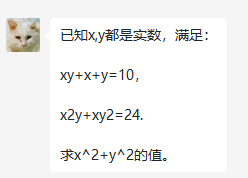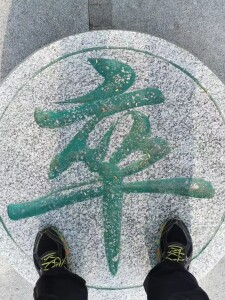# geogebra求解二元二次方程一例## 1. 题目

``````eq1: x*y + x + y = 10
eq2: x^(2) y + x y^(2) = 24``````

## 2. 解方程

``精确解({eq1,eq2},{x,y})``

``solve({eq1,eq2},{x,y})``

## 3. 验算

`element(element(solutions({eq1,eq2},{x,y}),1),1)^2+element(element(solutions({eq1,eq2},{x,y}),1),2)^2`

## 4． 再验算

``````x1:element(element(solutions({eq1,eq2},{x,y}),1),1)
y1:element(element(solutions({eq1,eq2},{x,y}),1),2)
x1^(2)*y1+x1*y1^(2)
x1*y1+x1+y1``````

``````x1:element( element(solve({eq1,eq2},{x,y}),1),1)
-> x = -sqrt(5) + 3
y1:element( element(solve({eq1,eq2},{x,y}),1),1)
-> y = sqrt(5) + 3``````

## 6. 复数解

x^2如果是正的，那么x就是实数。但是x^2+y^2是正的，可能x^2是负的（因此x不是实数），但是y^2是个更大的正数呢。

``csolve({eq1,eq2},{x,y})``

# 8.竞赛题